Test: Electrical Machines- 1

# Test: Electrical Machines- 1

Test Description

## 10 Questions MCQ Test RRB JE for Electrical Engineering | Test: Electrical Machines- 1

Test: Electrical Machines- 1 for Electrical Engineering (EE) 2022 is part of RRB JE for Electrical Engineering preparation. The Test: Electrical Machines- 1 questions and answers have been prepared according to the Electrical Engineering (EE) exam syllabus.The Test: Electrical Machines- 1 MCQs are made for Electrical Engineering (EE) 2022 Exam. Find important definitions, questions, notes, meanings, examples, exercises, MCQs and online tests for Test: Electrical Machines- 1 below.
Solutions of Test: Electrical Machines- 1 questions in English are available as part of our RRB JE for Electrical Engineering for Electrical Engineering (EE) & Test: Electrical Machines- 1 solutions in Hindi for RRB JE for Electrical Engineering course. Download more important topics, notes, lectures and mock test series for Electrical Engineering (EE) Exam by signing up for free. Attempt Test: Electrical Machines- 1 | 10 questions in 30 minutes | Mock test for Electrical Engineering (EE) preparation | Free important questions MCQ to study RRB JE for Electrical Engineering for Electrical Engineering (EE) Exam | Download free PDF with solutions
 1 Crore+ students have signed up on EduRev. Have you?
Test: Electrical Machines- 1 - Question 1

### A 50 KVA, 11000/400 V Δ-Y distribution transformer has a resistance of 1% and reactance of 7% per unit. What is the transformer phase impedance referred to the high-voltage side?

Detailed Solution for Test: Electrical Machines- 1 - Question 1

∴ Its base impedance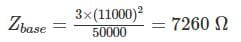The PU impedance of the transformer is
Zeq = [0.01 + j 0.07] PU
So the high-side impedance in ohm is
Zeq = Zeq pu × Zbase
= (0.01 + j0.07) × 7260 Ω
= 72.6 + j 508.2

*Answer can only contain numeric values
Test: Electrical Machines- 1 - Question 2

### An auto transformer is used to connect a 12.6 kV distribution line to a 13.8 kV distribution line. It must be capable of handling 2000 kVA. There are three phases, connected Y-Y with their neutrals solidly grounded. What must the NC/NSE­ turn ratio be to accomplish this connection? (NC→ common turn, NSE → Series turn)

Detailed Solution for Test: Electrical Machines- 1 - Question 2

The transformer is connected y-y so the primary and secondary phase voltage are the line voltage divided by 3–√3. The turn ratio of each auto transformer is given by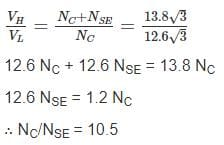Test: Electrical Machines- 1 - Question 3

### A 3-phase bank of three single phase transformer are fed from 3 phase 33 kV (L-L). It supplies a load of 5000 kVA at 11 kV (L-L). Both supply and load three wire. Calculate the voltage rating of single phase transformer for start-delta connection.

Detailed Solution for Test: Electrical Machines- 1 - Question 3

For star-delta connection
Primary side phase voltage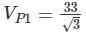Secondary side phase voltage = 11
∴ Transformer voltage rating 19.05/11 kV

Test: Electrical Machines- 1 - Question 4

Three single phase transformers are connected to form a3 - ϕ transformer bank the transformer are connected in the following manner.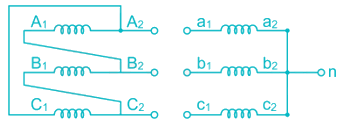the transformer connecting will be represented by

Detailed Solution for Test: Electrical Machines- 1 - Question 4

Phase diagram of primary and secondary voltage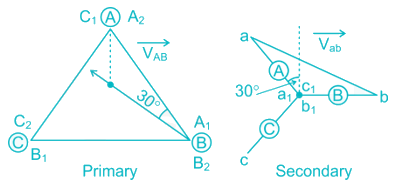this connection is known as Dy11 connectiong

*Answer can only contain numeric values
Test: Electrical Machines- 1 - Question 5

A 100,000-KVA 230/115 – kV Δ-Δ three phase power transformer has a per-unit resistance of 0.02 PU and a per unit reactance of 0.055. The excitation branch element are RC = 110 PU and XM = 20 PU. If this transformer supplies a load of 80 MVA at 0.85 P.F lagging. Find the voltage regulation (in %) of the transformer bank under these conditions.

Detailed Solution for Test: Electrical Machines- 1 - Question 5

The transformer supplies a load 80 MVA at 0.85 P.F lagging.

∴ Secondary line current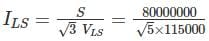= 402 A

The base value of the secondary line current is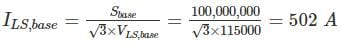So, the P.U secondary current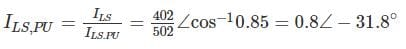The per unit primary voltage of this transformer
VP = VS + IZEQ = 1∠0° +0.8∠ − 31.8° (0.02 + j0.55)
= 1.037 ∠1.6°
The voltage regulation 1.037−11×100 = 3.7%

*Answer can only contain numeric values
Test: Electrical Machines- 1 - Question 6

The terminal voltage on the secondary side when supplying full load at 0.8 lagging P.F. is 440 V. the equivalent Resistance and reactance drops for the transformer are 1% and 5% respectively.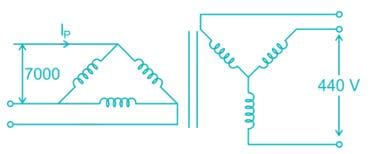Find Induced voltage per phase (in volt) at the secondary side.

Detailed Solution for Test: Electrical Machines- 1 - Question 6

Percentage voltage Regulation
= R cos ϕ + X sin ϕ
= 1 × 0.8 + 5 × 0.6 = 3.8%
We know that voltage regulation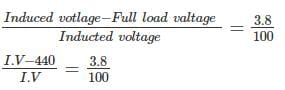I.V. – 440 = 0.038 I.V
I.V = 457.38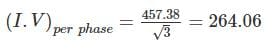*Answer can only contain numeric values
Test: Electrical Machines- 1 - Question 7

A1 ϕ 33 kVA, 3300 V/330 V, 50 Hz, distribution transformer is to be connected as an auto-transformer to get an output voltage of 3630 V. It maximum kVA rating as an auto-transformer is________kVA

Detailed Solution for Test: Electrical Machines- 1 - Question 7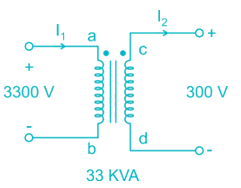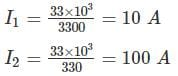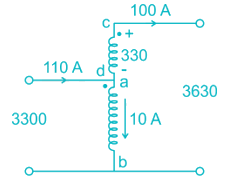Maximum kVA rating = V.A = VHIH = 3630 × 100 = 363 KVA

*Answer can only contain numeric values
Test: Electrical Machines- 1 - Question 8

A 50 Hz, 3ϕ core type star-delta transformer has a line valtage ratio of 11,000/440 volts. The cross-section of the core is square with a circumscribing circle of 0.5 m diameter. If maximum flux density of 1.30 ωb/m2 then find the number of turns per phase on high voltage windings. Assume insulation occupies 8% of the total core area.

Detailed Solution for Test: Electrical Machines- 1 - Question 8

We known that diameter of the circumscribing is same as diagonal of the square.
Area of square core = l × b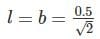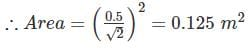∴ Net cross section area = 0.125 × 0.92 = 0.115
E.M.F/Turn = 4.44 fBA = 4.44 × 50 × 1.3 × 0.115 = 33.19
Phase turn ratio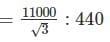∴ Number of turn per phase on high voltage side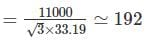Test: Electrical Machines- 1 - Question 9

In a Star\Delta 3 phase transformer connection, the turns ratio is N1/N2 with N1 being primary side turns and N2 being secondary side turns. What is the value of line voltage and current on secondary side, if the same for primary side are V & I respectively ?

Detailed Solution for Test: Electrical Machines- 1 - Question 9

line voltage at primary = V
Phase voltage at primary  =V/√3
Line current at primary = I
Phase current at primary = I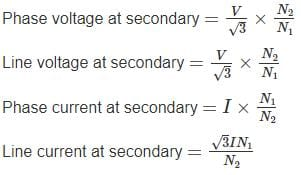Test: Electrical Machines- 1 - Question 10

A 3 – phase, Δ – Y, 33000 / 660 V, 150 kVA, 50Hz transformer is having resistance per phase of 4 Ω on primary side and 0.15 Ω on secondary side. If the efficiency of transformer and half load is 96.5% at 0.85 pf (lagging), then what are the iron losses?

Detailed Solution for Test: Electrical Machines- 1 - Question 10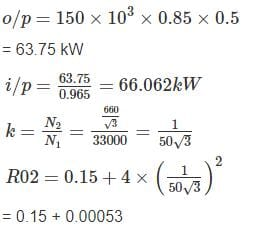= 0.15053 Ω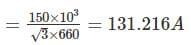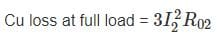= 3 × (131.216)2 × 0.15053
= 7,775.31 W
Cu loss at half load = (0.5)2 × 7775.31
= 1943.83 W
Losses = input - output
= 66062 – 63750
= 2312
Iron loss = total loss – cu loss
= 2312 – 1943.83
= 368.17 W

## RRB JE for Electrical Engineering

2 videos|3 docs|75 tests
 Use Code STAYHOME200 and get INR 200 additional OFF Use Coupon Code
Information about Test: Electrical Machines- 1 Page
In this test you can find the Exam questions for Test: Electrical Machines- 1 solved & explained in the simplest way possible. Besides giving Questions and answers for Test: Electrical Machines- 1, EduRev gives you an ample number of Online tests for practice

## RRB JE for Electrical Engineering

2 videos|3 docs|75 tests